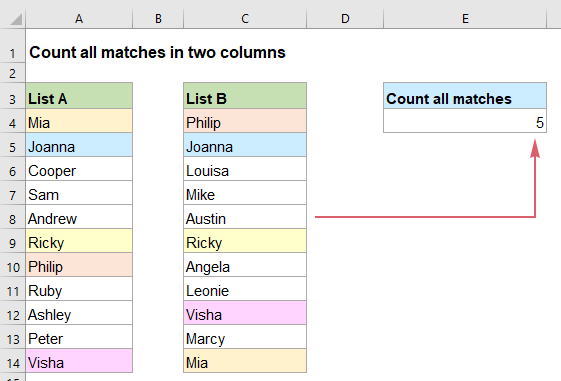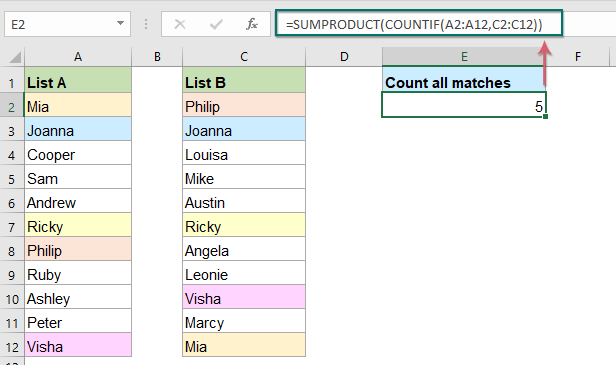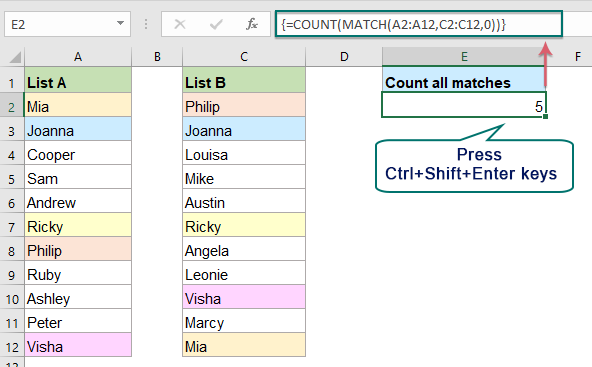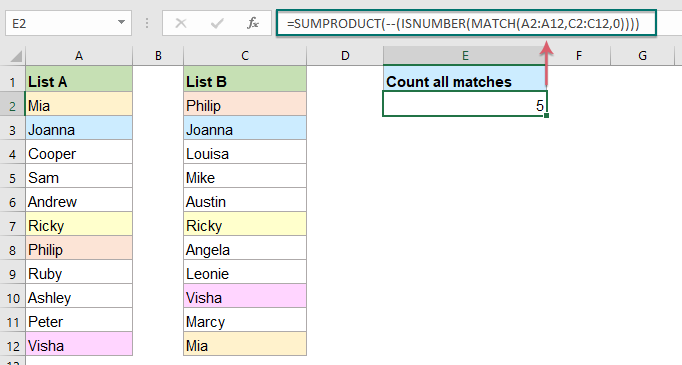## Count all matches / duplicates between two columns in Excel

Comparing two columns of data and count all matches or duplicates in the two columns may be a common task for most of us. For example, you have two columns of names, some names appear both in the first and second columns, now, you want to count all matched names (the matches located anywhere within the two columns) between two columns as below screenshot shown, this tutorial will introduce some formulas for achieving this goal in Excel.#### Count all matches between two columns with SUMPRODUCT and COUNTIF functions

To count all matches between two columns, the combination of SUMPRODUCT and COUNTIF functions can help you, the generic syntax is:

=SUMPRODUCT(COUNTIF(range1,range2))
• range1, range2: The two ranges contain the data you want to count all matches.

Now, please enter or copy the below formula into a blank cell, and then press Enter key to get the result:

=SUMPRODUCT(COUNTIF(A2:A12,C2:C12))##### Explanation of the formula:

=SUMPRODUCT(COUNTIF(A2:A12,C2:C12))

• COUNTIF(A2:A12,C2:C12): This COUNTIF function checks if each name from column C exists in column A. If the name exists, a number 1 is displayed, otherwise, a number 0 is displayed. The function will return the result as this: {1;1;0;0;0;1;0;0;1;0;1}.
• SUMPRODUCT(COUNTIF(A2:A12,C2:C12))=SUMPRODUCT({1;1;0;0;0;1;0;0;1;0;1}): The SUMPRODUCT function sums up all the items in this array and get the result: 5.

#### Count all matches between two columns with COUNT and MATCH functions

With the combination of COUNT and MATCH functions, you can also get the number of matches between two columns, the generic syntax is:

{=COUNT(MATCH(range1,range2,0))}
Array formula, should press Ctrl + Shift + Enter keys together.
• range1, range2: The two ranges contain the data you want to count all matches.

Please enter or copy the following formula into a blank cell, and then press Ctrl + Shift + Enter keys together to get the correct result, see screenshot:

=COUNT(MATCH(A2:A12,C2:C12,0))##### Explanation of the formula:

=COUNT(MATCH(A2:A12,C2:C12,0))

• MATCH(A2:A12,C2:C12,0): This MATCH function will look up the names from column A in column C, and return the position of each matched value. If a value is not found, an error value will be displayed. So, you will get the array list as this: {11;2;#N/A;#N/A;#N/A;6;1;#N/A;#N/A;#N/A;9}.
• COUNT(MATCH(A2:A12,C2:C12,0))= COUNT({11;2;#N/A;#N/A;#N/A;6;1;#N/A;#N/A;#N/A;9}): The COUNT function will count the numerals in the array list to get the result: 5.

#### Count all matches between two columns with SUMPRODUCT, ISNUMBER and MATCH functions

In Excel, you can try to find the matches in two columns and count then by using the SUMPRODUCT, ISNUMBER and MATCH functions, the generic syntax is:

=SUMPRODUCT(--(ISNUMBER(MATCH(range1,range2,0))))
• range1, range2: The two ranges contain the data you want to count all matches.

Enter or copy the below formula into a blank cell to output the result, and then press Enter key to return the calculation, see screenshot:

=SUMPRODUCT(--(ISNUMBER(MATCH(A2:A12,C2:C12,0))))##### Explanation of the formula:

=SUMPRODUCT(--(ISNUMBER(MATCH(A2:A12,C2:C12,0))))

• MATCH(A2:A12,C2:C12,0): This MATCH function will look up the names from column A in column C, and return the position of each matched value. If the value is not found, an error value will be displayed. So, you will get the array list as this: {11;2;#N/A;#N/A;#N/A;6;1;#N/A;#N/A;#N/A;9}.
• ISNUMBER(MATCH(A2:A12,C2:C12,0))= ISNUMBER({11;2;#N/A;#N/A;#N/A;6;1;#N/A;#N/A;#N/A;9}): Here, the ISNUMBER function converts the numerals to TRUE and other values to FALSE in the array. So, you will get an array like this: {TRUE;TRUE;FALSE;FALSE;FALSE;TRUE;TRUE;FALSE;FALSE;FALSE;TRUE}.
• --(ISNUMBER(MATCH(A2:A12,C2:C12,0)))=--({TRUE;TRUE;FALSE;FALSE;FALSE;TRUE;TRUE;FALSE;FALSE;FALSE;TRUE}): --this double negative sign is used to convert a TRUE value to 1 and a False value to 0 and returns the result as this: {1;1;0;0;0;1;1;0;0;0;1}.
• SUMPRODUCT(--(ISNUMBER(MATCH(A2:A12,C2:C12,0))))=SUMPRODUCT({1;1;0;0;0;1;1;0;0;0;1}): Finally, the SUMPRODUCT function will sum up all the items in this array and get the result: 5.

#### Relative function used:

• SUMPRODUCT:
• The SUMPRODUCT function can be used to multiply two or more columns or arrays together, and then get the sum of products.
• COUNTIF:
• The COUNTIF function is a statistical function in Excel which is used to count the number of cells that meet a criterion.
• COUNT:
• The COUNT function is used to count the number of cells that contain numbers, or count the numbers in a list of arguments.
• MATCH:
• The Microsoft Excel MATCH function searches for a specific value in a range of cells, and returns the relative position of this value.
• ISNUMBER:
• The ISNUMBER function returns TRUE when a cell contains a number, and FALSE if not.

#### More articles:

• Count Matches Between Two Columns
• For example, I have two lists of data in column A and column C, now, I want to compare the two columns and count if the value in column A found in column C in the same row as below screenshot shown. In this case, the SUMPRODUCT function may be the best function for you to solve this task in Excel.
• Count Number Of Cells That Contain Specific Text In Excel
• Supposing, you have a list of text strings, and may want to find the number of cells that contain certain text as part of their contents. In this case, you can use the wildcard characters (*) that represents any texts or characters in your criteria when applying the COUNTIF function. This article, I will introduce how to use formulas for dealing with this job in Excel.
• Count number of cells not equal to many values in Excel
• In Excel, you may easily get the number of cells not equal to a specific value by using the COUNTIF function, but have you ever tried to count the cells which are not equal to many values? For example, I want to get the total number of the products in column A but exclude the specific items in C4:C6 as below screenshot shown. This article, I will introduce some formulas for solving this job in Excel.

### The Best Office Productivity Tools

#### Kutools for Excel - Helps You To Stand Out From Crowd

 Popular Features: Find, Highlight or Identify Duplicates  |  Delete Blank Rows  |  Combine Columns or Cells without Losing Data  |  Round without Formula ... Super VLookup: Multiple Criteria  |  Multiple Value  |  Across Multi-Sheets  |  Fuzzy Lookup... Adv. Drop-down List: Easy Drop Down List  |  Dependent Drop Down List  |  Multi-select Drop Down List... Column Manager: Add a Specific Number of Columns  |  Move Columns  |  Toggle Visibility Status of Hidden Columns  |  Compare Columns to Select Same & Different Cells ... Featured Features: Grid Focus  |  Design View  |  Big Formula Bar  |  Workbook & Sheet Manager | Resource Library (Auto Text)  |  Date Picker  |  Combine Worksheets  |  Encrypt/Decrypt Cells  |  Send Emails by List  |  Super Filter  |  Special Filter (filter bold/italic/strikethrough...) ... Top 15 Toolsets:  12 Text Tools (Add Text, Remove Characters ...)  |  50+ Chart Types (Gantt Chart ...)  |  40+ Practical Formulas (Calculate age based on birthday ...)  |  19 Insertion Tools (Insert QR Code, Insert Picture from Path ...)  |  12 Conversion Tools (Numbers to Words, Currency Conversion ...)  |  7 Merge & Split Tools (Advanced Combine Rows, Split Excel Cells ...)  |  ... and more

Kutools for Excel Boasts Over 300 Features, Ensuring That What You Need is Just A Click Away...#### Office Tab - Enable Tabbed Reading and Editing in Microsoft Office (include Excel)

• One second to switch between dozens of open documents!
• Reduce hundreds of mouse clicks for you every day, say goodbye to mouse hand.
• Increases your productivity by 50% when viewing and editing multiple documents.
• Brings Efficient Tabs to Office (include Excel), Just Like Chrome, Edge and Firefox.